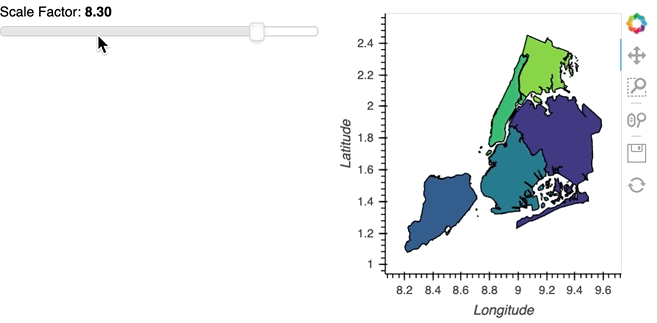# Update data from gv.Polygons

Hello,
I wonder how to update data from gv.Polygons without rerendering all in panel ?

With this base,
I want to change Unemployment by another dataset (here random) dynamically without reloading all.

``````from bokeh.sampledata.us_counties import data as counties
from bokeh.sampledata.unemployment import data as unemployment

from random import random

cts = [dict(county, Unemployment=unemployment[cid])
for cid, county in counties.items()
if county["state"] == "tx"]

rdm = [dict(county, random=100* random())
for cid, county in counties.items()
if county["state"] == "tx"]

choropleth = gv.Polygons(cts, ['lons', 'lats'], [('detailed name', 'County'), 'Unemployment']).opts(
colorbar=True, toolbar='above', xaxis=None, yaxis=None)

tiles = StamenTerrain()
tiles * choropleth
``````

What would be the most efficient way to do it ?

I take this simple exemple but the real one is there: https://github.com/slamer59/fragilite_num_playground/blob/7e57426028f54110d5e09ac62a7ed39b858e8391/mednum/medapp.py#L173

In what I construct, I have function plot in class that returns tiles * choropleth . On the left, I will filter values so I always rerender all which is not really good (it blinks, takes times, …)

2 Likes

To take advantage of more optimized updates you will want to use a HoloViews `DynamicMap` which returns the updated data, as an example here we assign some random values to a geopandas dataframe in the callback depending on the value of a widget:

``````import geopandas as gpd
import geoviews as gv
import numpy as np
import cartopy.crs as ccrs

gv.extension('bokeh')

polygons = polygons.to_crs(epsg=3857)

def update_poly(some_value):
polygons['value'] = np.random.rand(5) * some_value

widget = pn.widgets.FloatSlider(name='Scale Factor', start=1, end=10)

pn.Row(
widget,
gv.tile_sources.Wikipedia() * gv.DynamicMap(pn.bind(update_poly, widget))
)
``````1 Like

One thing in particular you should avoid is returning the tiles in the callback and overlay them separately since that ensures it is static and does not flicker.

Thanks for quick feed back.

Never try bind function but looks promising. Tried hv.DynmaicMap but without success.

You mention the recreation of the tile but in your exemple gv.Polygons is also recreated from scratch. Might be interesting to change only the dataset and update values only. Feasible ?

Since I am using bokeh backend, I saw patching capabilities in Bokeh which seems to do this, but not sure it is easy to access Bokeh object.
https://docs.bokeh.org/en/latest/docs/user_guide/data.html#patching

Or maybe with streams ? http://holoviews.org/user_guide/Plots_and_Renderers.html

And for the tile, definitely yes, it was quick & dirty.Recreating the objects from scratch doesn’t actually indicate anything about what data is synced, since the entire HoloViews model means that the objects are declarative and immutable. So the answer is yes, but that should all happen internally inside HoloViews and a user shouldn’t have to worry about it. If you’re familiar with the React JS framework you should think of HoloViews being similar in that you return a declarative object and it figures out a performant bokeh model update internally just like React computes efficient DOM updates. Certainly there are many cases where this needs to be further optimized but that’s the idea.

So please describe what you tried concretely with DynamicMaps (ideally with code), so we can figure out what’s going wrong. To be concrete on my end, in your code, the relevant line in the view method should look something like this:

``````pn.Column(self.top_panel, pn.Spacer(height=80), tiles * hv.DynamicMap(self.plot)),
``````

Any other way of triggering this refresh of the polygons without necesarily binding it to a widget? Can callbacks trigger the map refresh? I’m trying something similar with points and changes are not reflected in the map until I rerun the cell or refresh the page where I’m serving the map.

Here I present an example where I try to change the color of a point when I change the value of a property on an object. The callback actually works, but the map doesn’t register the change until I refresh

``````#Minimum reproducible example
import panel as pn
import param
import geoviews as gv
from cartopy import crs

class Example(param.Parameterized):
loc = param.XYCoordinates(None)
num = param.Integer()

@property
def color(self):
if self.num > 0:
return 'green'
else:
return 'red'

a = Example(num=5, loc=(262953, 4802244))
b = Example(num=-3, loc=(262653, 4802344))
tiles = gv.tile_sources.OSM

def plot_example(example):
data ={'x': example.loc,
'y': example.loc,
}

point = gv.Points(data, kdims=['x', 'y'],
crs=crs.UTM(30)).opts(size=15, color=example.color)

def update_point(event):
if event.name == 'num':
point.opts(color='blue')

watcher = example.param.watch(update_point,['num'])

return point, watcher

p_a, w_a = plot_example(a)
p_b, w_b = plot_example(b)

final_map = tiles * p_a * p_b
final_map
pn.Row(final_map).show()

a.num= -22 # Here I just try to trigger the change

``````

This callback and object refreshing method is taken from this thread regarding indicators (what got me into this discourse).

Although I present an example without a DynamicMap, casting those points into a dynamic map doesn’t work for me either, as I’m not actually changing the inputs for the object to dynamically refresh.

Any way to easily refresh the map in this instance?

Did you find a solution to DynamicMap not working for you? Any other alternatives accesing bokeh directly?

@CesarRodriguez
I am still having issue. The exemple of Philipp works good but I have a problem of focus on a map when I am changing of locations.

I have two things to control. The first in my question is answered. How I change the values at the same location and how to change of location.

The second part is failing to have the proper Latitude and Longitude. For what I understand tiles controls this (since it’s the first member of * operation).

For now, I don’t have a minimal example to show why it’s not working for me.

``````import geoviews as gv
import panel as pn
from geoviews import opts
from cartopy import crs as ccrs
import param

import geopandas as gpd
import geoviews as gv
import numpy as np
import cartopy.crs as ccrs

gv.extension("bokeh")

polygons = polygons.to_crs(epsg=3857)

class Example(param.Parameterized):
localisation = param.ObjectSelector(
default="Queens", objects=list(polygons.BoroName)
)
some_value = param.Integer(bounds=(0, 100))
tiles = gv.tile_sources.StamenTerrain()

@pn.depends("some_value", "localisation")
def update_poly(self):
poly = polygons.copy()
poly = poly[poly.BoroName == self.localisation]

poly.loc[:, "value"] = np.random.rand(len(poly)) * self.some_value